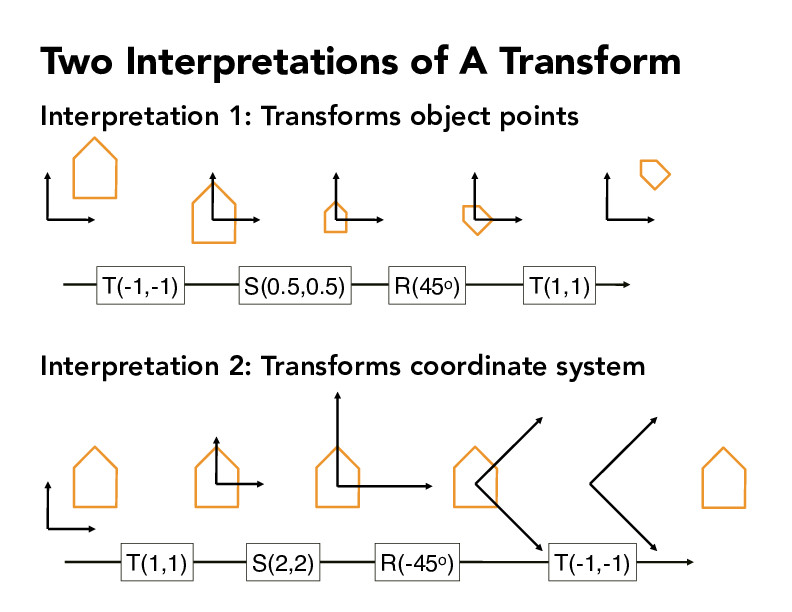Lecture 4: Transforms (42)hangg7

This slide to me illustrates an important concept that in practice we should always pay attention to what we are transforming, i.e. are we transforming an object or a coordinate. Because what we usually care about is the relative relation between the object and coordinate.

Examples could be helpful:

• We want to transform object, when we are modeling a moving object while assuming a static camera (including "world" camera).
• We want to transform coordinate, when we are modeling a static object but from a different view. So the object remain the same xyz position in world coordinate.

Here I have another question related to this: suppose we have two transforms parametrized by 4x4 matrices: $M_1$ applying to object, and $M_2$ applying to coordinate, they result in same relative position (meaning the xyz position of each point on the object within that coordinate remain the same). What is the mathematic relation between $M_1$ & $M_2$? -- $M_1$ = $M_2^{-1}$?ziyaointl

Yeah that's some good intuition! I believe you are right: $M_1 = M_2^{-1}$.yirenng

@hangg7 Thank you for the thoughtful comment. One small comment -- our CS184 website allows you to typeset comments in Markdown LaTeX. I edited your comment to write your matrix variables using Markdown. You can learn more in this short article about using Markdown in our CS184 website, and we encourage you and all students to use these features to enhance your comments going forward!

You must be enrolled in the course to comment